# Rewriting Expressions using Math Laws

Previously, you learned about converting words to equations and expressions. You now know the difference between expression and equation.
In this lesson, you will learn about rewriting expressions, using mathematical fundamental laws.

If two expressions are written differently, are they equal ?

That is the kind of question we are trying to answer here. I assume that you know basic addition, and multiplication.

## Mathematical Laws

To ensure that expression written in different forms are equal, we will test them against mathematical laws. These laws applies to expressions involving addition and multiplication. These are:

• Commutative law
• Associative law
• Distributive law

## Commutative Law

Let me discuss the Commutative laws. Let a, b and c be three whole numbers, and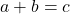is the expression.

The commutative laws states that the order of addition does not affect the result. It means thatis equivalent to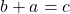.

You add two number in any order, the result is going to be same. This is resulting from commutative law.

### Example

Our first example is a simple equation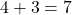which is same as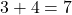. Both results in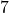.

4 + 3 = 3 + 4 = 7

Commutative law for Multiplication

If we multiply two number in any order , the result will be same which means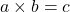is equivalent to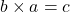. So, commutative laws applies to multiplication as well.

Let’s consider another example,

Suppose 5 and 3 are two whole numbers, if we multiply 5 with 3, the answer is 15. Let us rearrange the expression into 3 multiplied by 5 which is also 15. therefore, commutative law is true for multiplication as well.

5 \times 3 = 3 \times 5 = 15

## Associative Laws

The associative laws works on addition and multiplication. If three or more numbers are added or multiplied, different groupings of numbers does not affect the results.

If a, b and c are three numbers. Then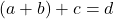is same as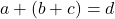.

### Example:

let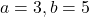, and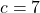, then

\begin{aligned}
&(a + b ) + c = (3 + 5) + 7 \\\\
& Evaluate \hspace{3px} first \hspace{3px}  3 + 5 = 8\\\\
&8 + 7 = 15
\end{aligned}

Now we must evaluate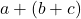\begin{aligned}
&a + (b + c) = 3 + (5 + 7) \\\\
&Evaluate \hspace{3px} first \hspace{3px}5 + 7 = 12\\\\
&3 + 12 = 15
\end{aligned}

Our expression becomes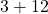which is equal to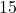. so, by associative law addition of three numbers with different grouping is equal and does not affect the results.

Associative Law for Multiplication

The different grouping does not affect multiplication as well. You can multiply variable by grouping them in any order and it still gives same results.

Let a, b and c be three numbers multiplied together. (a multiplied by b) multiplied by c is equal to d. similarly, a multiplied by (b multiplied by c) is also equal to d, where d is a whole number.

(a \times b ) \times c = (b \times a ) \times c = d

Let us see an example of associativity of multiplication.

### Example:

(2 \times 6) \times 5 = 2 \times  (6 \times 5)

In the left hand expression, we must evaluate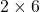first which is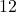and then multiply with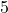which gives us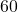.

\begin{aligned}
&(2 \times 6) \times 5\\\\
&=12 \times 5 \\\\
&=60
\end{aligned}

Similarly, in the right hand side expression, we must evaluate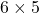first which is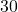and then multiplied by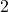will give us.

\begin{aligned}
&2 \times (6\times 5)\\\\
&=2 \times 30 \\\\
&=60
\end{aligned}

Therefore, the equation is true and associative law is true. You can rewrite any expression using the commutative and associative laws.

## Identity Law

Next I will talk about another law called the identity law. This mathematical law applies to addition and multiplication only. The identity law requires identity element in the expression. The identity element for addition is 0 and the identity element for multiplication is 1.

If a is whole number, then according to identity law,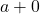where 0 is the identity element gives the same number a as result.

By commutative law,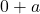is also equal to a.

a + 0 = 0 + a = a

I illustrated this with an example below.

### Example:

Let us see an example, suppose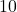is a whole number , then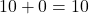. Also,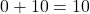.

Identity Law for Multiplication

For multiplication, let a be any whole number multiplied by its identity element 1, then

a \times 1 = a

we get number a itself. By commutative law,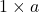is also, the number a..

### Summary

Let me summarize what you learned.

• Commutative law says, if a and b are two whole numbers then
\begin{aligned}
&a + b = b + a\\\\
&a \times b = b \times a
\end{aligned}
• The associative law says , if a, b, and c are 3 numbers,then
\begin{aligned}
&(a + b) + c = a + (b + c)\\\\
&( a \times b ) \times c = a \times ( b \times c )
\end{aligned}
• The identity element involve the identity element. The identity element for addition is 0 and 1 is identity element for multiplication. Then,
\begin{aligned}
&a + 0 = 0 + a = a\\\\
&a \times 1 = 1 \times a = a
\end{aligned}

Note: examples in this article uses only two or three variables, however, if you try any of these examples with more than three variables, the result would be true only. The commutative, associative and identity laws applied to expressions with more variables.But, for simplicity I have chosen fewer than two or three variables.